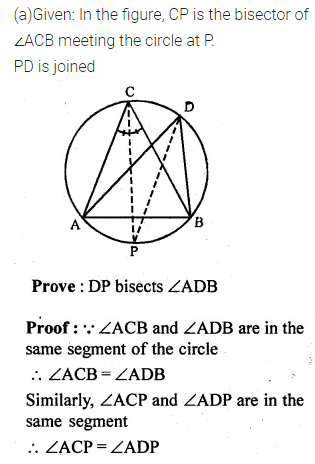Guru

# Question 16. (a) In the figure (i) given below, CP bisects ∠ACB. Prove that DP bisects ∠ADB. (b) In the figure (ii) given below, BDbisects ∠ABC. Prove that AB/BD=BE/BC

• 1

This is an important and exam oriented question from Chapter name- circles
Topic – Angle properties of circles
Chapter number- 15

In this ques we have a figure and given that CP bisects ∠ACB. Now we have to prove that DP bisects ∠AD

And (b) it is given that BD bisects ∠ABC. Now we have to Prove that AB/BD=BE/BC
ICSE Avichal publication
Understanding ICSE Mathematics
Question 16

Share